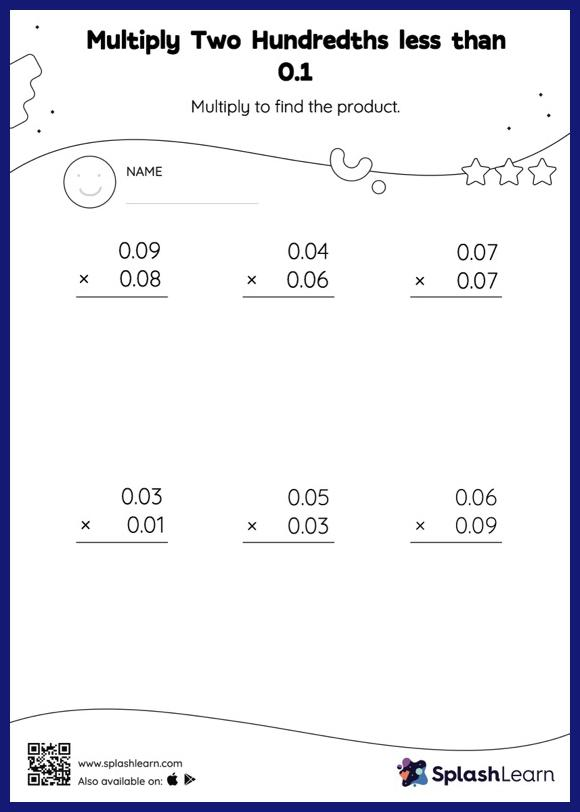# Multiply Two Hundredths less than 0.1: Vertical Multiplication Worksheet

Home > Multiply Two Hundredths less than 0.1: Vertical MultiplicationStudents first multiply as though there is no decimal when multiplying two decimals. They then add the same number of digits after the decimal point in the product as there are decimal digits in each number. This multiply two hundredths less than 0.1 Worksheet gives students a thorough practice of this concept. As the worksheet uses the column method, it is helpful in getting students toward higher accuracy, especially with bigger numbers and in scenarios where regrouping is required.